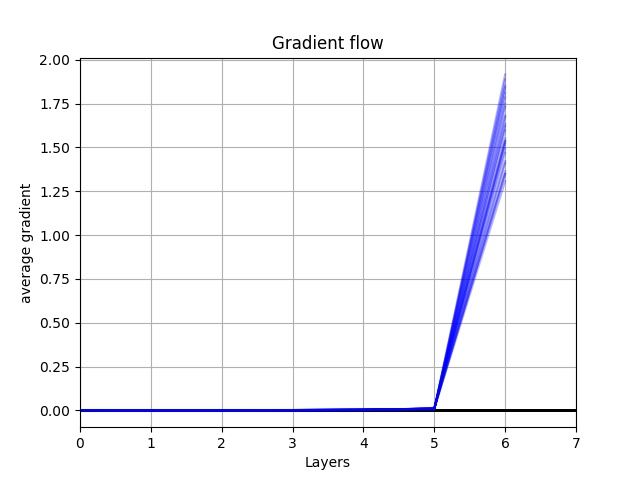# Why by adding second loss and applying the back.ward(), generated gradient is as same as before?

Hi There,
I add the second loss to my first loss and apply backward.(), but the gradient results after backward.() is as same as when I used just one loss. The first loss is nn.BCELoss() and the second loss is L1. I try different second loss , but does not have effect. It seems that backward.() does not create any gradient on the second loss regardless of type. I check the gradient flow just for the second loss which was 0. I am wondering if you have any ideas.

``````optimizerG = optim.Adam(netG.parameters(), lr=lr2, betas=(beta1, 0.999))
netG = Generator994(ngpu,nz,ngf).to(device)

## -----------------------------------------------------
for p in netG.parameters():

label.fill_(real_label)
label=label.to(device)
output = netD(fake).view(-1)
# Calculate G's loss based on this output
loss1 = criterion(output, label)
hist_rGau= np.histogram(Gaussy.squeeze(1).view(-1).detach().numpy(), bins=FFBins,range=[0 ,1])
count_r11 = hist_rGau
PGAUSSY=count_r11/(count_r11.sum())
hist_rGAN = np.histogram(fake.squeeze(1).view(-1).detach().numpy(), bins=FFBins,range=[0 ,1])
count_r22 = hist_rGAN
PGAN=count_r22/(count_r22.sum())

## -------- L1 loss  and second loss---------------------------
loss2=abs(PGAUSSY-PGAN).sum()

## ----- total loss --------------------------
loss= loss1+loss2

loss.backward()

for param in netG.parameters():
optimizerG.step()

## --------------------------------------------------------
class Generator994(nn.Module):
def __init__(self,ngpu,nz,ngf):
super(Generator994, self).__init__()
self.ngpu=ngpu
self.nz=nz
self.ngf=ngf
self.l1= nn.Sequential(
# input is Z, going into a convolution
nn.ConvTranspose2d(self.nz, self.ngf * 8, 3, 1, 0, bias=False),
nn.BatchNorm2d(self.ngf * 8),
nn.ReLU(True),)

self.l2=nn.Sequential(nn.ConvTranspose2d(self.ngf * 8, self.ngf * 4, 3, 1, 0, bias=False),
nn.BatchNorm2d(self.ngf * 4),
nn.ReLU(True),)

self.l3=nn.Sequential(nn.ConvTranspose2d( self.ngf * 4, self.ngf * 2, 3, 1, 0, bias=False),
nn.BatchNorm2d(self.ngf * 2),
nn.ReLU(True),)

self.l4=nn.Sequential(nn.ConvTranspose2d( self.ngf*2, 1, 3, 1, 0, bias=False),nn.Sigmoid()

)

def forward(self, input):
out=self.l1(input)
out=self.l2(out)
out=self.l3(out)
out=self.l4(out)
return out``````

You are detaching the computation graph by using numpy functions for `loss`:

``````hist_rGau= np.histogram(Gaussy.squeeze(1).view(-1).detach().numpy(), bins=FFBins,range=[0 ,1])
count_r11 = hist_rGau
PGAUSSY=count_r11/(count_r11.sum())
hist_rGAN = np.histogram(fake.squeeze(1).view(-1).detach().numpy(), bins=FFBins,range=[0 ,1])
count_r22 = hist_rGAN
PGAN=count_r22/(count_r22.sum())

loss2=abs(PGAUSSY-PGAN).sum()
``````

If you need to use numpy operations, you would need to implement the `backward` function manually via a custom `autograd.Function` or use PyTorch operations.

Double post from:
here, here, here, here, here.
Please don’t repost the same question in 6 different threads, as this will only waste the time of community members, which might be looking into your issue.

I am really sorry, I am a bit stress and though no one see my post. Please accept my apology.
Regarding the code, I used torch and no detach and just focus on the second loss (loss2) it gives me
AttributeError: ‘NoneType’ object has no attribute ‘data’)

I think it creates no gradient again.

``````        for p in netG.parameters():

label.fill_(real_label)
label=label.to(device)
output = netD(fake).view(-1)
# Calculate G's loss based on this output
xxx=torch.histc(Gaussy.squeeze(1).view(-1).cpu(),100, min=0, max=1, out=None)
ddGaussy=xxx/xxx.sum()

xxx1=torch.histc(fake.squeeze(1).view(-1).cpu(),100, min=0, max=1, out=None)
ddFake=xxx1/xxx1.sum()

loss2=abs(ddGaussy-ddFake).sum().item()

## ----- total loss --------------------------
loss= loss2

loss.backward()
for param in netG.parameters():
optimizerG.step()

``````

I don’t think that `histc` is differentiable without an approximation, so you could follow this topic for potential workarounds.

Ptrblck,

I am really confuse. I try to use the L1 from pytorch package not mine, and forget about numpy, again gives me the same error , would you please help me more with that. I would appreciate your time.

(AttributeError: ‘NoneType’ object has no attribute ‘data’)
Is there any thing wrong with my model? or layers? weights initialization?

``````      criterion2=nn.L1Loss()

for p in netG.parameters():

label.fill_(real_label)
label=label.to(device)
output = netD(fake).view(-1)

ddGaussy=xxx/xxx.sum()

xxx1=torch.histc(fake.squeeze(1).view(-1).cpu(),100, min=0, max=1, out=None)
ddFake=xxx1/xxx1.sum()

MSECMBSS=criterion2(ddFake,ddGaussy)

## ----- total loss --------------------------

loss2.backward()

for param in netG.parameters():

optimizerG.step()
``````

I tried the JS loss as well. but for all types it gave me same error. After detach I used Variable and requires_grad too. How is it possible non of them work! and give same error

``````        MSECMBSS=jensenshannon(ddFake.detach().numpy(),ddGaussy.detach().numpy())**2
``````

orr

``````  loss = SamplesLoss(loss="sinkhorn", p=1, blur=.5)
ccc=fake.cpu().squeeze(1).view(-1,81)
ccc1=Gaussy.cpu().squeeze(1).view(-1,81)

L1=geomloss.SamplesLoss()(ccc.unsqueeze(2),ccc1.unsqueeze(2))
MSECMBSS=L1.sum().item()
``````

If you recreate a new tensor, you will break the computation graph:

``````Variable(MSECMBSS,requires_grad=True)
# and
``````

Also, calling `detach()` will detach this tensor from the computation graph and Autograd won’t calculate the gradients for any parameters which were used to create this tensor. The same applies to `numpy()` calls.

Note that `Variables` are deprecated since `0.4` so you shouldn’t use them anymore.

To keep the computation graph complete, you would have to only use PyTorch methods, without any calls to `detach()`, `numpy()`, `item()`, or recreating tensors.

1 Like

I really appreciate your patience and time.
Yes you are right . I just removed the item() from the last code and it works for me.
I think it is a good code for others to use.

Be careful, if you’ve just removed the `item()` operation in this code snippet:

``````L1=geomloss.SamplesLoss()(ccc.unsqueeze(2),ccc1.unsqueeze(2))
MSECMBSS=L1.sum().item() # HERE
``````

as you would be still be recreating the tensor, which will not create any gradients in the model.

``````L1 = geomloss.SamplesLoss()(ccc.unsqueeze(2),ccc1.unsqueeze(2))
MSECMBSS = L1.sum().item()
loss2 = MSECMBSS
``````

I used this:

``````        L1=geomloss.SamplesLoss()(ccc.unsqueeze(2),ccc1.unsqueeze(2))
MSECMBSS=L1.sum()
loss2 = MSECMBSS
loss2.backward.()
``````

here is the flow of the gradient. I think just last layer have gradient and the earliest layer doe snot have. the numbers are very small.Do you think the gradient is good? the first layers does not have any gradient changes.

Small gradients would be a different issue than no gradients at all.
If the `.grad` attributes in the layers 0 to 4 are `None`, the computation graph would still be detached at some point. On the other hand, if the gradients are just small e.g. the model architecture might lower the gradient magnitude.

1 Like

You means that it is better to reduce the number of layers ? to have more considerable gradient?
The activation function in the last layer is Sigmoid and middles are ReLU, with batch norm. Do you think
BatchNorm2d can have effect on gradients?